## TMDL Report Selection Tool

please cite as: Kumar, S. (2016). TMDL Report Selection Tool. Retrieved curdate, from https://occviz.com/tmdl

3
I need to download 6MB dataset. loading ...This (no-name yet!) app is being developed by Saurav Kumar ( https://sites.google.com/site/kumarsaurav/ email: kumar.saurav+tmdl@gmail.com). Drop me a note if you find this useful or to suggest changes.

## How to use this app

This app assists with filtering the table of TMDL reports based on your interests. There are two steps to using this app:

1. Select the "critical frequency" using the slider on the top right of this page.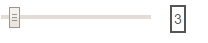This slider controls how reports are classified as having used one or more models. For example, if the selected value for the slider is "30", then any model name (and its derivatives or alternates; see notes on regex later) has to occur 30 or more times in the text of that report, for the report to be marked as using that model. Notice as you move the slider to a higher number, fewer reports are available. This slider has no impact on impairment, as impairment labels were included in the metadata about the report from EPA and assumed to be correctly classified.
2. Click on the groups in the chord graph to filter reports and investigate relationships. Click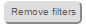button anytime to clear all filters.

## Data generation

1. Most of the data were obtained from USEPA ATTAINS database from the URL  http://iaspub.epa.gov/apex/waters/f?p=131:72:::NO:RP:P72_REGION,P72_LEAD_STATE,P72_POLLUTANT_GROUP,P72_FISCAL_YEAR_ESTABLISHED,P72_SEARCH_TERMS:99,XX,XX,XX,tmdl.
2. The textual contents of the reports obtained from USEPA ATTAINS (pdfs) were searched for occurrences of models covered by ASCE EWRI TMDL task committee using following "regex". Use  https://regex101.com/#python to test these regex; note the text after the colon is the regex.
` "mass balance":"(?i)mass(.{0,1}\s{0,1})(?i)balance(.{0,1}\s{0,1})(?i)model","simple method":"(?i)simple(.{0,1}\s{0,1})((?i)method|(?i)model)","bathub":"(\s|\(|\))(?i)bathub(\s|\(|\))","sstemp":"(\s|\(|\))(?i)sstemp(\s|\(|\))","load duration curve":"(((?i)load|(?i)flow)(.{0,1}\s{0,1})(?i)duration(.{0,1}\s{0,1})(?i)curve|\sldc\s)","usle":"(\s|\(|\))(USLE|RUSLE)(\s|\(|\)|\d)","agnps":"(\s|\(|\)|(?i)ann(-{0,1}|\s{0,1}))(?i)agnps(\s|\(|\))","answers":"(\s|\(|\))ANSWERS(\s|\(|\)|-)","dwsm":"(\s|\(|\))(?i)dwsm(\s|\(|\))","gssha":"(\s|\(|\))(?i)gssha(\s|\(|\))","gwlf":"(\s|\(|\))((?i)gwlf|(?i)basinsim)(\s|\(|\)|-)","hec-hms":"(\s|\(|\))(?i)hec(\s|-)(?i)hms(\s|\(|\)|v|V)","hspf":"(\s|\(|\))((?i)hspf|(?i)lspc)(\s|\(|\))","basins":"(\s|\(|\))BASINS(\s|\(|\)|-)","kineros":"(\s|\(|\))(?i)kineros(\s|\(|\)|-)","mike she":"(\s|\(|\)|)(?i)mike(.{0,1}\s{0,1})(?i)she(\s|\(|\))","stepl":"(\s|\(|\))(?i)stepl(\s|\(|\)|-)","spreadsheet":"(\s|\(|\))(?i)spreadsheet(\s|\(|\)|-)","swat":"(\s|\(|\))(?i)swat(\s|\(|\)|-)","swmm":"(\s|\(|\)|-)(?i)swmm(\s|\(|\)|-)","warmf":"(\s|\(|\))(?i)warmf(\s|\(|\)|-)","curve number":"(\s|\(|\))(?i)curve(.{0,1}\s{0,1})(?i)number(\s|\(|\)|-)","cche":"(\s|\(|\))(?i)cche(\s|\(|\)|-)","cequalriv":"(?i)ce(.{0,1}\s{0,1})(?i)qual(.{0,1}\s{0,1})(?i)riv","cequalw2":"(?i)ce(.{0,1}\s{0,1})(?i)qual(.{0,1}\s{0,1})(?i)w2","concepts":"(\s|\(|\))CONCEPTS(\s|\(|\)|-|,)","efdc":"(\s|\(|\))(?i)efdc(\s|\(|\)|-|,)","edp riv1":"(\s|\(|\))(?i)edp(.{0,1}\s{0,1})(?i)riv", # riv has number"hec ras":"(\s|\(|\))(?i)hec(.{0,1}\s{0,1})(?i)ras(\s|\(|\))","mike 11":"(\s|\(|\))(?i)mike(.{0,1}\s{0,1})(?i)11(\s|\(|\))","minteq":"(\s|\(|\))((?i)mineql|(?i)minteq)", # no end space "oteq": "(\s|\(|\))(?i)oteq(\s|\(|\))","qual":"(\s|\(|\))(?i)qual(.{0,1}\s{0,1})(?i)2e(\s|\(|\))","qual":"(\s|\(|\))(?i)qual(.{0,1}\s{0,1})(?i)2k(\s|\(|\))","wasp":"(\s|\(|\))(?i)wasp(\s|\(|\)|-)","sustain":"(\s|\(|\))SUSTAIN(\s|\(|\)|-)","tidal prism":"(\s|\(|\))(?i)tidal(.{0,1}\s{0,1})prism(\s|\(|\))"`

## What we have-- a quick look at data

1) There were 20,537 records that I got from EPA ATTAINS database. This included various types of documents.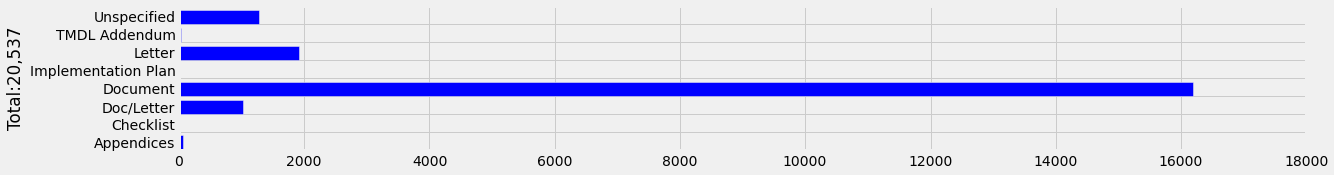2) I was able to filter records marked as "Document" down to 1891 unique TMDL development and implementation reports. The filtering procedure was based on manual filtering along with clues from the pdf see https://github.com/skp703/tmdl/blob/master/tmdl_scraper.ipynb. These 1891 reports were used for further analysis. Summary of the reports: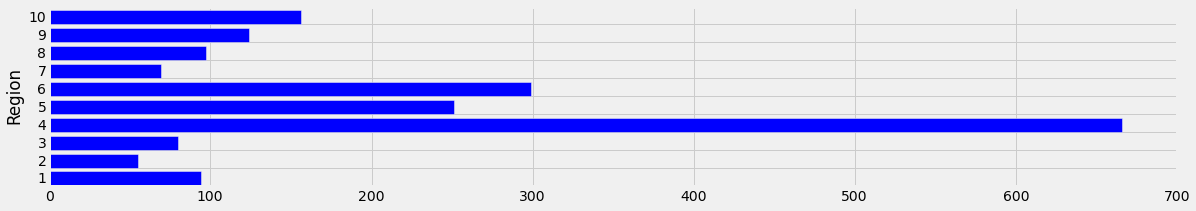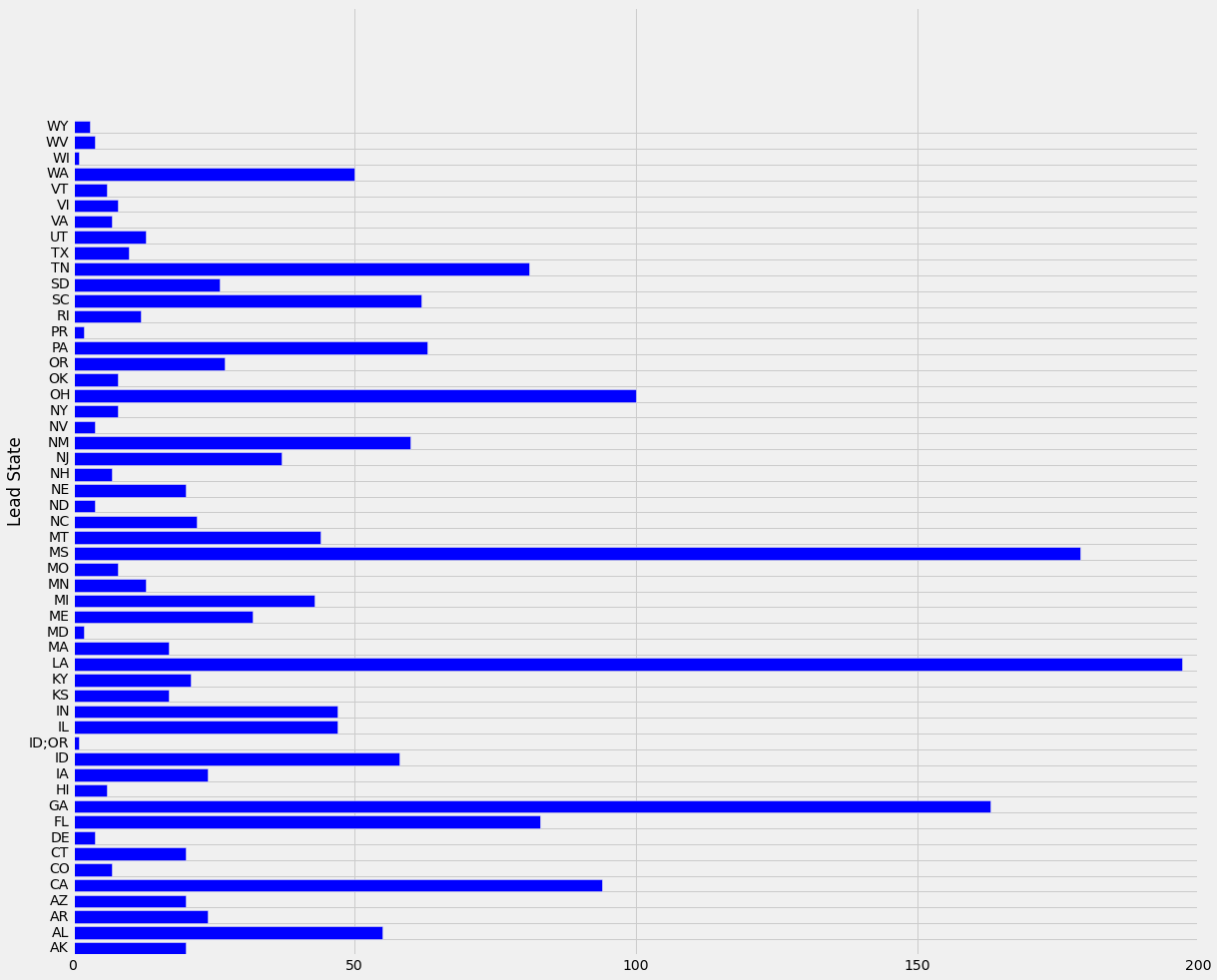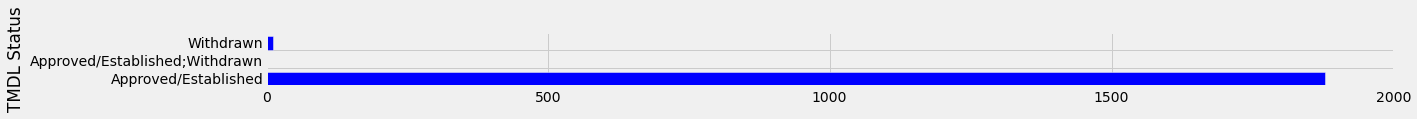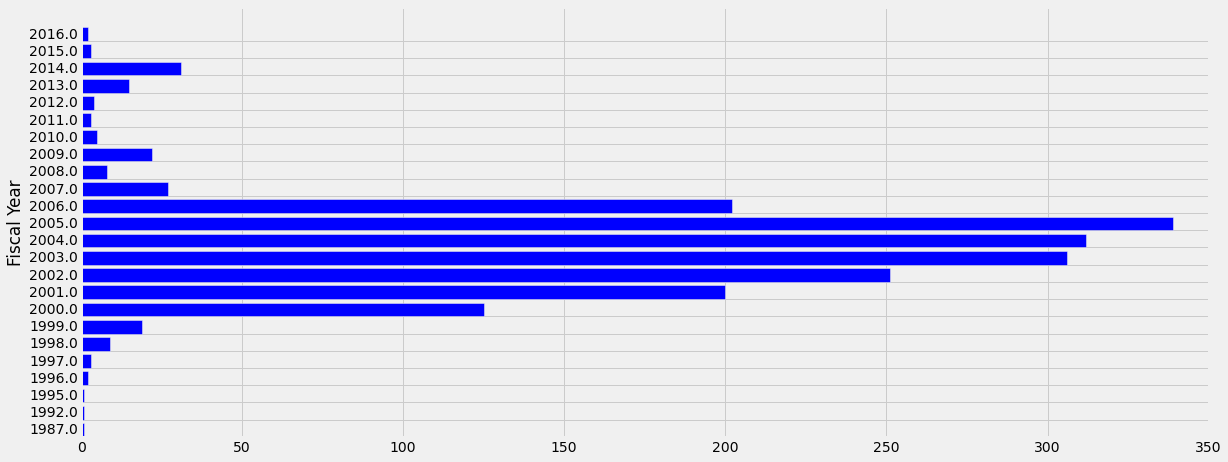For more details see (HTML PAGE) https://github.com/skp703/tmdl/blob/on_github/tmdl_scraper.ipynb and https://github.com/skp703/tmdl

Raw data in excel format is at https://docs.google.com/viewer?a=v&pid=sites&srcid=ZGVmYXVsdGRvbWFpbnxrdW1hcnNhdXJhdnxneDo1YTI2YTI0NjY1NGNhZGQ2